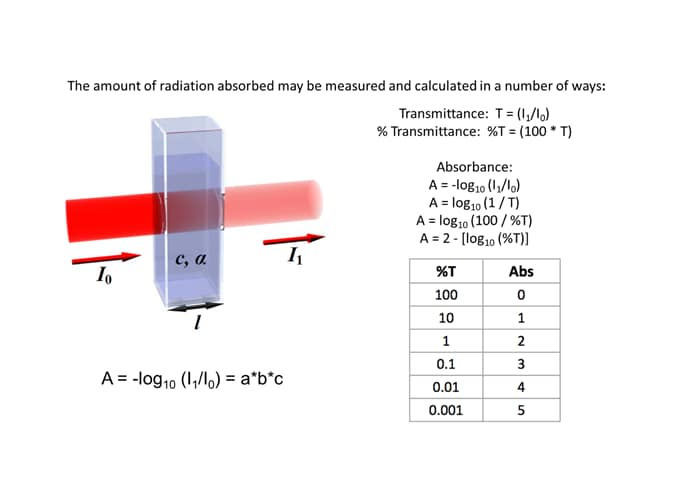# How does a spectrophotometer measure a sample?For each wavelength of light passing through the spectrometer, the intensity of the light passing through the sample cell is measured. The intensity of light entering the sample is usually referred to as I0. The intensity of the light passing through the sample cell and emerging on the other side is usually designated I1. If we now calculate the percent of the ratio these two values (I1/I0)*100 we get a percent transmission (%T) value. The %T value is a measure of the light that passes through the sample to strike the detector. If I1 is less than I0, then obviously the sample has absorbed some of the incident light. A simple bit of mathematics is then done in the computer to convert this into something called the absorbance of the sample, given the symbol, A (sometimes abs). The absorbance is a measure of the amount of light that disappears (interacts) with the sample. The Beer-Lambert Law (above), is the relationship between absorbance as a function of sample concentration c, the sample extinction coefficient a, the  sample path length L, I0, and I1 is given by:

For most inexpensive spectrophotometers you will come across, the absorbance ranges from 0 to 3, but it can go higher than that for more expense instruments. The UV-3600i Plus and Solid Spec 3700i can measure up to 8 and sometimes higher. An absorbance of 0 means that no light of that particular wavelength has been absorbed by the sample. The intensity of light entering and exiting the sample are both the same, so the ratio I1/I0 is 1, so -log10 of 1 is zero. An absorbance of 1 happens when 90% of the light at that wavelength has been absorbed, which means that the intensity is 10% of what it would otherwise be. In that case, I1/I0 is 10/100 = 0.1) and -log10 of 0.1 is 1.

Absorbance is a logarithmic scale similar to the Richter Scale used for earthquakes. An increase of 1 unit on the absorbance scale translates into a factor of 10 decrease on the %T scale. Another name people tend to use for a logarithmic relationship (factor of 10 change) is “order of magnitude”. Thus an instrument that can measure to 3 absorbance units has a range of 3 orders of magnitude. Remember also, as the %T value decreases the absorbance value increases. Above is a simple table that summarizes the relationship between %T and absorbance.

Back to Index